- 将图片的内容转化为单个整数(a single integer).

 - 基于计算得到的哈希值，处理图片数据集中的相同或者接近相同的图片.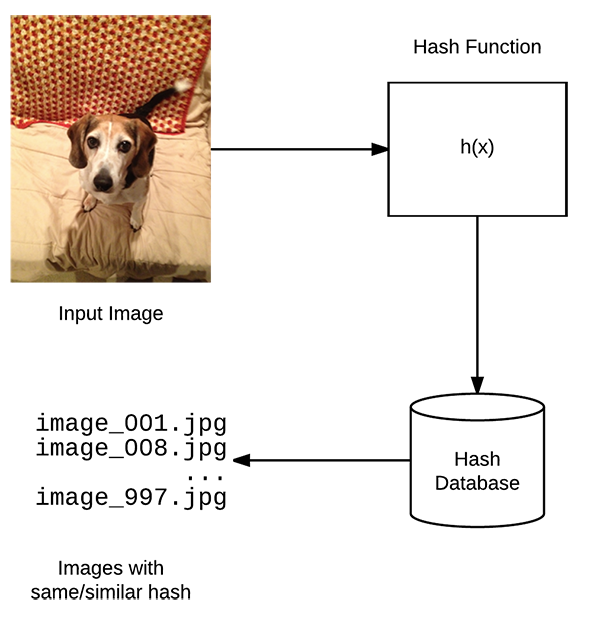## 1. 图片哈希

Image hashing with OpenCV and Python - 2017.11.27

 - 检查图片的内容；

 - 基于图片的内容来构建哈希值，以唯一的表征输入图片.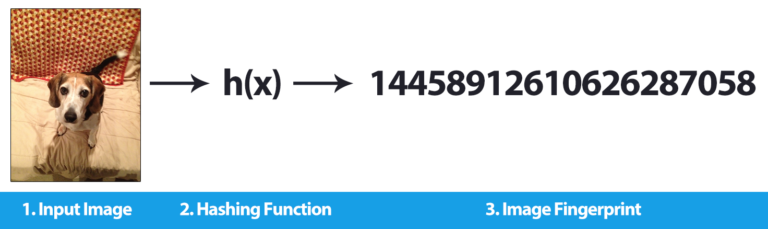- 介绍图片哈希和感知哈希；

 - 图片哈希的实现，尤其是 dHash(difference hashing).

 - 采用图片哈希处理实际问题和数据集.

### 1.1. md5 哈希

Ubuntu - md5sum 文件校验工具 - AIUAI

md5 也是用于计算和校验文件的.

identify test.png
md5 test.png
convert test.png -resize 250x test_resize.png
md5 test_resize.png

### 1.2. dHash

dHash 大致包含四步处理，相对直接和易于理解.

 - 将图片转化为灰度图(grayscale)

(1)-哈希计算速度更快，因为只需要在一个通道上进行计算.

(2)-图片相似性匹配时减少颜色空间的变化的影响.

 - 调整灰度图尺寸(resize)

(1)-为什么在 resize 时忽略图片的长宽比？ —— 确保相似图片不受其初始空间维度的影响.

(2)-为什么是 9x8？(后面会解释)

 - 计算差值(difference)

dHash 旨在计算 64-bit 的哈希值，采用 8x8=64 更接近与此. 但为什么将灰度图尺寸调整为 9x8 呢？

 - 构建哈希值(hash)

dHash 的最后一步处理是字节分配和构建哈希值.

### 1.3. dHash 的特点

dHash 有很多优点，基本特点包括：

 - 即使输入图像的长宽比发生变化，图片哈希值也不会改变.

 - 调整亮度(brightness) 或者对比度(contrast)，其一般不会改变图片哈希值. 即使发生轻微变化，也能保证相似图片的哈希值比较接近.

 - dHash 计算速度快(extremely fast).

### 1.4. dHashes 对比

HackerFactor 中建议哈希值间的 Hamming 距离 >10 bits 则两张图片是不同的，如果哈希值在 1-10 bits 则可以认为两张图片是相同或相似的. 实际中可以根据应用场景和数据集特点调整 Hamming 距离阈值.

### 1.5. dHash 实现

from imutils import paths
import time
import sys
import cv2
import os

def dhash(image, hashSize=8):
image = cv2.cvtColor(image, cv2.COLOR_BGR2GRAY)
#调整输入图片尺寸
resized = cv2.resize(image, (hashSize + 1, hashSize))

#计算临近列像素间的相对水平梯度.
diff = resized[:, 1:] > resized[:, :-1]

#差值图片转化为哈希值
return sum([2 ** i for (i, v) in enumerate(diff.flatten()) if v])

## 2. 图片搜索引擎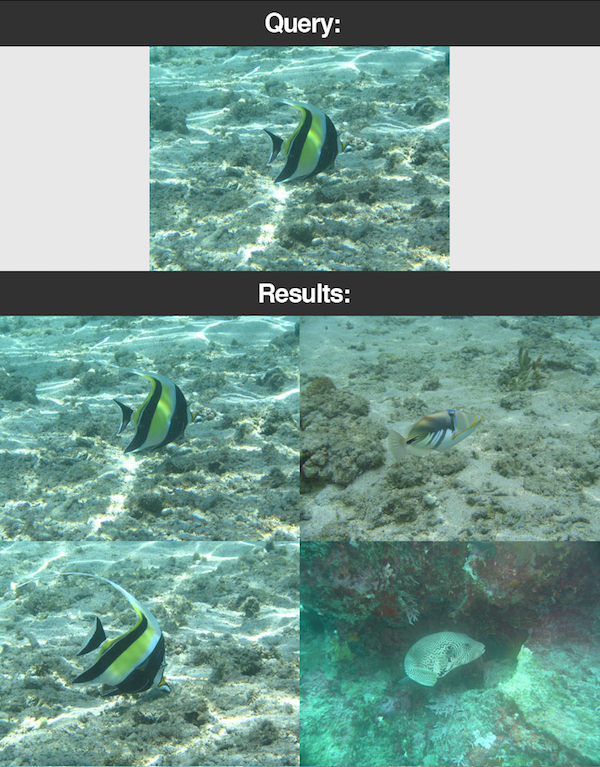### 2.1. 图片哈希表示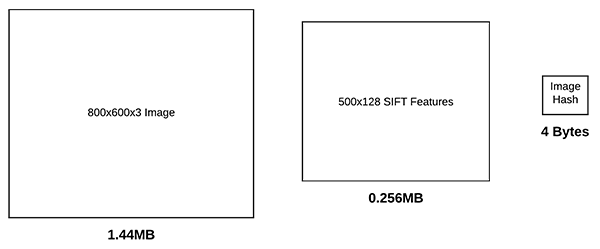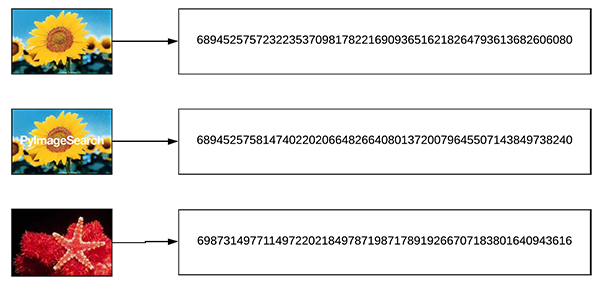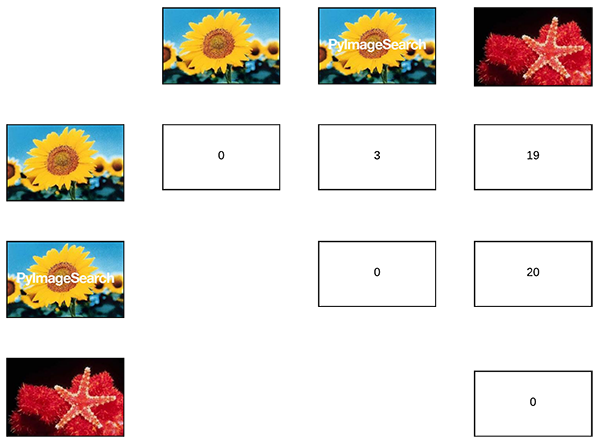### 2.2. 图片哈希搜索引擎

 - 索引(Indexing)

 - 搜索/查询(Searching/Querying)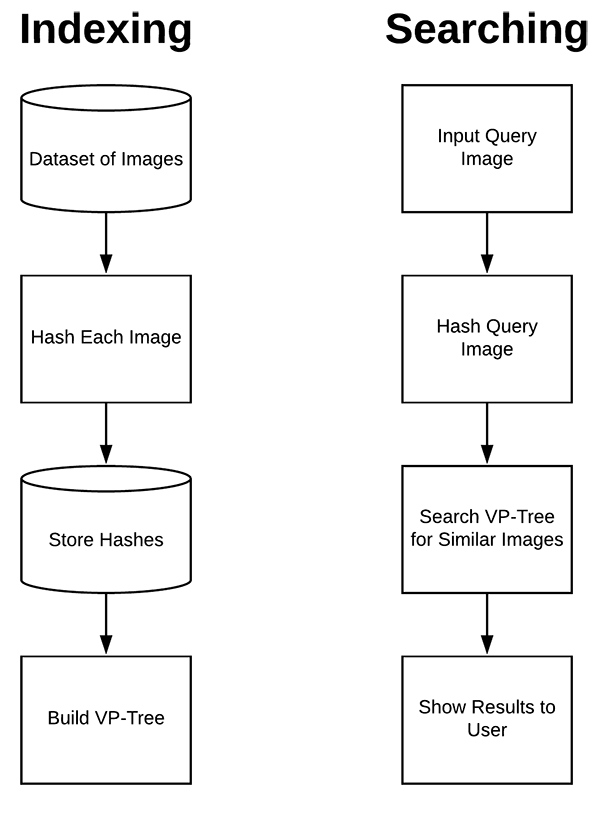### 2.3. 面临的问题

 - 已有一个 1000000 张图片的数据集；

 - 已经分别计算每张图片的图片哈希；

 - 用户提供一张输入图片，然后要求查询数据集中所有相似图片.

### 2.4. VP-Tree 数据结构

 - 减少搜索的线性复杂度(linear complexity), O(n) ...

 - 降低到 sub-linear complexity，如理想值 O(log n) ...

VP-Trees 是一种在度量空间进行操作的度量树，通过选择度量空间中的一个给定位置，如有利点(vantage point)，将数据点划分为两个数据子集：

• 靠近 vantage point 的数据点；
• 远离 vantage point 的数据点.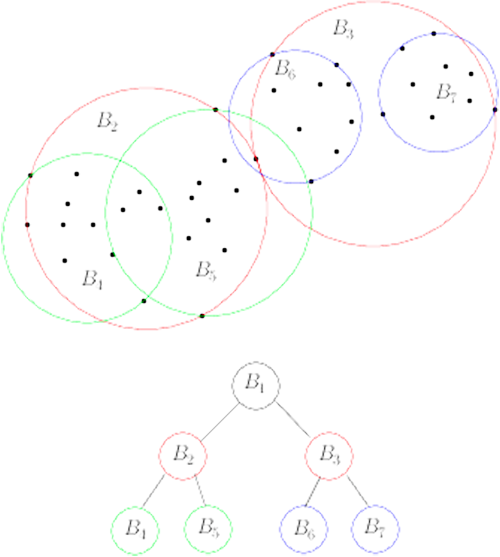VP-Tree 的构建过程如图：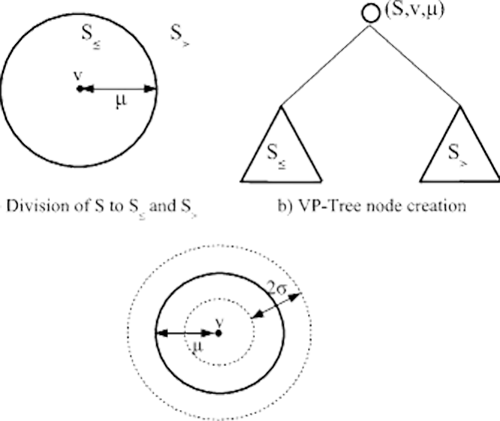• 距离小于等于 $\mu$ 的所有点，属于 $S1$;
• 距离大于 $\mu$ 的所有点，属于 $S2$.

• 其距离都较小；
• 相比于树中其它数据点，其更相似.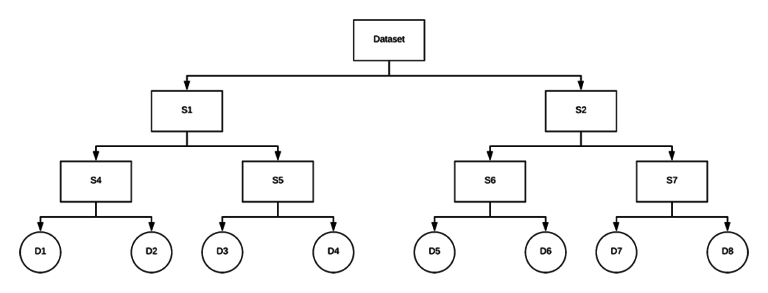VP-Trees 构建的复杂度为 O(nlogn)，但是仅需要构建一次；而单词查询的复杂度只需 O(logn)，即，将搜索时间复杂度降低到了 sub-linear complexity.

## 3. 图片哈希搜索引擎 Python 实现

 - 图片哈希相关辅助函数 - hashing.py

import numpy as np
import cv2

def dhash(image, hashSize=8):
gray = cv2.cvtColor(image, cv2.COLOR_BGR2GRAY)
resized = cv2.resize(gray, (hashSize + 1, hashSize))
diff = resized[:, 1:] > resized[:, :-1]

return sum([2 ** i for (i, v) in enumerate(diff.flatten()) if v])

def convert_hash(h):
#将哈希值转换为 numpy 的 64-bit 浮点数；
#然后再转换为 int 格式.
return int(np.array(h, dtype="float64"))

def hamming(a, b):
#计算 Hamming 距离
return bin(int(a) ^ int(b)).count("1")

 - CALTECH-101 图片数据集，共 101 个类别，9144 张图片，每个类别大概有 40-800 张图片.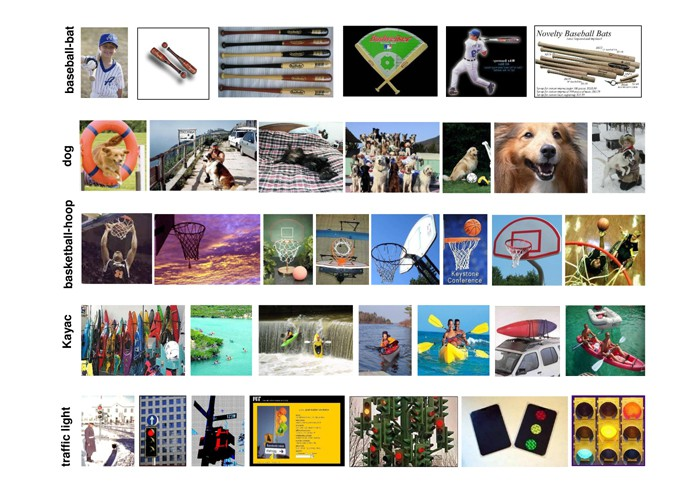### 3.1. 图片数据集索引(Index)

index_image.py:

from hashing import convert_hash
from hashing import hamming
from hashing import dhash
from imutils import paths
import pickle
import vptree
import cv2

#
imagePaths = list(paths.list_images("/path/to/CALTECH-101/images/"))
hashes = {}

# loop over the image paths
for (i, imagePath) in enumerate(imagePaths):
print("[INFO] processing image {}/{}".format(i + 1,
len(imagePaths)))

#计算图片哈希值，并转化数据格式
h = dhash(image)
h = convert_hash(h)

#update the hashes dictionary
l = hashes.get(h, [])
l.append(imagePath)
hashes[h] = l

#构建VP-Tree
print("[INFO] building VP-Tree...")
points = list(hashes.keys())
tree = vptree.VPTree(points, hamming)

#将 VP-Tree 序列化写入到磁盘
print("[INFO] serializing VP-Tree...")
f = open("/path/to/output/vptree.pickle", "wb")
f.write(pickle.dumps(tree))
f.close()

#将哈希值序列化到字典
print("[INFO] serializing hashes...")
f = open("/path/to/output/hashes.pickle", "wb")
f.write(pickle.dumps(hashes))
f.close()

### 3.2. 图片查询(Search)

search.py:

from hashing import convert_hash
from hashing import dhash
import pickle
import time
import cv2

#加载 VP-Tree 和哈希字典

#读取输入的查询图片
cv2.imshow("Query", image)

#计算查询图片的哈希值，并转换数据格式
queryHash = dhash(image)
queryHash = convert_hash(queryHash)

#查询
print("[INFO] performing search...")
start = time.time()
#maximum_hamming_distance - 相似度阈值
results = tree.get_all_in_range(queryHash, "maximum_hamming_distance")
results = sorted(results)
end = time.time()
print("[INFO] search took {} seconds".format(end - start))

# loop over the results
for (d, h) in results:
# grab all image paths in our dataset with the same hash
resultPaths = hashes.get(h, [])
print("[INFO] {} total image(s) with d: {}, h: {}".format(
len(resultPaths), d, h))

# loop over the result paths
for resultPath in resultPaths:
cv2.imshow("Result", result)
cv2.waitKey(0)

## 4. 相关材料

 - PyImageSearch Gurus

 - VP Tree - Ivan Chen

 - 相似图片搜索的原理 - 阮一峰

 - 相似图片搜索的原理（二）- 阮一峰

Last modification：September 6th, 2019 at 01:05 pm